# GSEB Class 9 Maths Notes Chapter 10 Areas of Parallelograms and Triangles

This GSEB Class 9 Maths Notes Chapter 10 Areas of Parallelograms and Triangles covers all the important topics and concepts as mentioned in the chapter.

## Areas of Parallelograms and Triangles Class 9 GSEB Notes

Circle and its related terms:
Circle:
The collection of all the points in a plane, which are at a fixed distance from a fixed point in the plane, is called a circle.
The fixed point is called the centre of the circle and the fixed distance is called the radius of the circle. In the given figure, P is the centre of the circle and the length of PA is called the radius of the circle.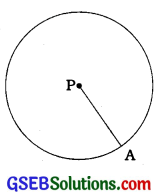The line segment joining the centre and any point on the circle is also called a radius of the circle.
Note: The word ‘radius’ is used in two senses : line segment PA and the length of line segment PA.

Partition of the plane by a circle: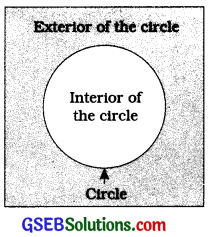A circle divides the plane on which it lies into three parts:

1. Inside the circle, which is also called the interior of the circle.
2. Circle and
3. Outside the circle, which is also called the exterior of the circle. The circle and its interior make up the circular region.

Chord of a circle:
If you take any two points on a circle, then the line segment joining them is called a chord of the circle.In the given figure, AB is a chord.

Diameter of a circle:
The chord which passes through the centre of the circle is called a diameter of the circle.
In the given figure, XY is a diameter of the circle. Just like radius, the word ‘diameter’ is used in two senses: line segment XY and the length of line segment XY. Diameter is the longest chord. All diameters have the same length which is equal to two times the radius.Arc of a circle:
A piece of a circle between two points on a circle is called an arc of the circle.
In the given figure, two parts of the circle are received by points P and Q on the circle, one longer and the other shorter. The longer part is called major arc PQ and the shorter part is called minor arc PQ. The minor arc PQ is also denoted by $$\widehat{\mathrm{PQ}}$$ and the major arc PQ by $$\widehat{\mathrm{PRQ}}$$, where R is some point on the arc between P and Q.Unless otherwise stated, arc PQ or $$\widehat{\mathrm{PQ}}$$ stands for minor arc PQ. When P and Q are ends of a diameter, then both arcs are equal and each is called a semicircle.

Circumference:
The length of the complete circle is called its circumference.

Segment of a circle:
The region between a chord and either of its arcs is called a segment of the circular region or simply a segment of the circle.
The segment formed by a chord and a minor arc is called a minor segment, while / the segment formed by a chord and a major arc is called a major segment.Sector of a circle:
The arc and the two radii, to the end points of the arc is called a sector.A sector formed by a minor arc and radii through its end points is called a minor sector, while a sector formed by a major arc and radii through its end points is called a major sector. When two arcs are equal, i.e., each is a semicircle, then both the sectors and both the segments become the same and each is known as a semicircular region.Angle subtended by a chord at a point:
Angle subtended by a line segment at a point: Given a line segment and‘a point not on the line containing that line segment, the angle formed by joining p that exterior point to the end points of the line segment is called the angle subtended by that line segment at that point.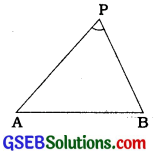In the given figure, point P lies outside the line segment AB. By joining P to A and P to B, Z APB is formed which is called the angle subtended by AB at R

Angles subtended by a chord: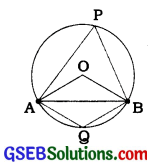AB is a chord of the circle with centre O. P is a point on major arc AB and Q is a point on minor arc AB.
Here, ZAOB is the angle subtended by chord AB at the centre O, Z APB is the angle subtended by chord AB at point P of the major arc and ZAQB is the angle subtended by chord AB at point Q of the minor arc.

Theorem 10.1:
Equal chords of a circle (or of congruent circles) subtend equal angles at the centre (or corresponding centres).

Theorem 10.2:
If the angles subtended by the chords of a circle (or of congruent circles) at the centre (corresponding centres) are equal, then the chords are equal.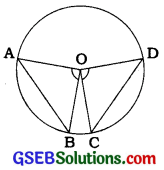Both the above theorems are converse of each other. If AB = CD is given, it prove that ∠AOB = ∠COD and if ∠AOB = ∠COD is given, it proves that AB = CD.

Perpendicular from the centre to a chord:
Theorem 10.3:
The perpendicular from the centre of a circle to a chord bisects the chord.

Theorem 10.4:
The line drawn through the centre of a circle to bisect a chord is perpendicular to the chord.
Above two theorems are converse of each other.

An important result:
The perpendicular bisector of any chord of a circle passes through the centre of the circle.

Circle through three points:
Infinitely many circles pass through a point given in a plane. Similarly, infinitely many circles pass through two points given in a plane.

Theorem 10.5:
There is one and only one circle passing through three given non-collinear points.
Note; Given a triangle ABC, according to theorem 10.5, there is one and only one circle passing through all the vertices A, B and C of A ABC. This circle is called the circumcircle of A ABC,, the centre of this circle and its radius are called circumcentre and circumradius of ∆ ABC.

Example 1:
Given an arc of a circle, complete the circle.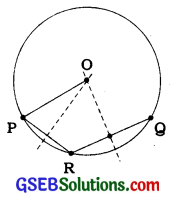Let arc PQ of a circle be given. Take a point R on the arc. Join PR and RQ. Draw the perpendicular bisectors of PR and RQ to intersect each other at O. Then, O will the centre of the required circle. Now, draw a circle with centre O and radius OP In this manner, a circle can be completed from its given arc. (See the given figure)

Distance of a line from a point: Let AB be a line and P be a point not on AB. From R ‘ draw perpendicular PM to AB, where point M lies on AB. The length of this perpendicular segment PM is called the distance of line AB from point E It is also called the distance of point P from line AB. If point P lies on line AB, then its distance from the line is zero.

Equal chords and their distances from the centre :
Theorem 10.6: Equal chords of a circle (or of congruent circles) are equidistant from the centre (or centres).
Theorem 10.7: Chords equidistant from the centre (or corresponding centres) of a circle (or of congruent circles) are equal in length.

Example 1:
If two intersecting chords of a circle make equal angles with the diameter passing through their point of intersection, prove that the chords are equal.
Given that AB and CD are two chords of a circle, with centre O intersecting at a point E. PQ is a diameter through E, such that ∠AEQ = ∠DEQ (see the given figure). Draw perpendiculars OL and OM on chords AB and CD, respectively.Now,
∠ LOE = 180° – 90° – ∠LEO (Angle sum property of a triangle)
= 90° – ∠ LEO
= 90° – ∠AEQ
= 90° – ∠DEQ
= 90° – ∠MEO
= ∠ MOE

In A OLE and A OME,
∠LEO = ∠MEO (Given ∠AEQ = ∠DEQ)
∠LOE = ∠MOE (Proved above)
EO = EO (Common)
∴ ∆OLE = ∆OME (ASA rule)
∴ OL = OM (CPCT)
∴ AB = CD (Theorem 10.7)
Note: To prove ∆OLE ≅ ∆OME, we can use ∠LEO = ∠MEO, ∠OLE = ∠OME (Right angles), EO = EO and AAS rule as well.Angle subtended by an arc of a circle:
If two chords of a circle are equal, then their corresponding arcs are congruent and conversely, if two arcs are congruent, then their corresponding chords are equal.

Angle subtended by an arc at the centre:
Angle subtended by an arc at the centre is defined to be the angle subtended by the corresponding chord at the centre. Minor arc AB subtends ∠AOB at the centre and major arc AB subtends reflex ∠AOB at the centre.Congruent arcs (or equal arcs) of a circle subtend equal angles at the centre.

Theorem 10.8:
The angle subtended by an arc at the centre Is double the angle subtended by It at any point on the remaining part of the circle.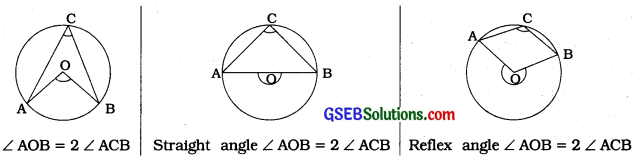Note: ∠ACB is also called an angle in segment ACBA. Angle in a semicircle is a right angle.

Theorem 10.9:
Angles in the same segment of a circle are equal.Theorem 10.10:
If a line segment joining two points subtends equal angles at two other points lying on the same side of the line containing the line segment, the four points lie on a circle (i.e., they are concyclic).

A quadrilateral is called a cyclic quadrilateral if all the four vertices of it lie on a circle.

Theorem 10.11:
The sum of either pair of opposite angles of a cyclic quadrilateral is 180°.

Theorem 10.12:
If the sum of a pair of opposite angles of a quadrilateral is 180°, the quadrilateral is cyclic.
Thus, if quadrilateral ABCD is cyclic, then ∠A + ∠C = ∠B + ∠D = 180° and if in quadrilateral ABCD, ∠A + ∠C = ∠B + ∠D = 180°, then ABCD is cyclic.
Note: In quadrilateral ABCD, ∠A + ∠C = 180° automatically gives ∠B + ∠D = 180°.

Example 1:
In the given figure, AB is a diameter of the circle, CD is a chord equal to the radius of the circle. AC and BD when extended intersect at a point E. Prove that ∠AEB = 60°.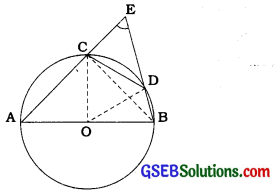Join OC, OD and BC.
Triangle ODC is an equilateral triangle.
(OC = OD = CD = radius)
∴ ∠COD = 60°
Now, ∠CBD = ∠COD (Theorem 10.8)
∴ ∠CBD = 30°
Again, ∠ACB = 90° (Angle in a semicircle)
So, ∠BCE = 180° – ∠ACB = 90°
∴ In ∆ CBE, ∠ CEB = 90° -30° = 60°,
i. e., ∠ AEB = 60°

Example 2:
In the given figure, ABCD is a cyclic s quadrilateral in which AC and BD are its diagonals. If ∠ DBC = 55° and ∠ BAC = 45°, find ∠ BCD.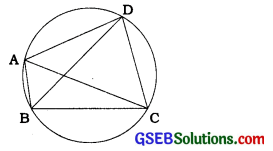∠ CAD = ∠ DBC = 55° (Angles in the same segment)
Then, ∠ DAB = ∠ CAD + ∠ BAC = 55° + 45° = 100°
Now, ∠ DAB + ∠ BCD = 180° (Opposite angles of a cyclic quadrilateral)
∴ ∠ BCD =180°- 100° = 80°

Example 3:
Two circles intersect at two points A and B. AD and AC are diameters to the two circles (see the given figure). Prove that B lies on the line segment DC.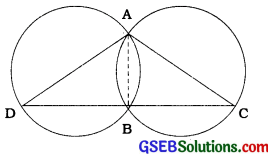Join AB.
∠ ABD = 90° (Angle in a semicircle)
∠ ABC = 90° (Angle in a semicircle)
So, ∠ ABD + ∠ ABC = 90° + 90° = 180°
Therefore, DBC is a line, i.e., B lies on the line segment DC.Example 4:
Prove that the quadrilateral formed (if possible) by the internal angle bisectors of any quadrilateral is cyclic.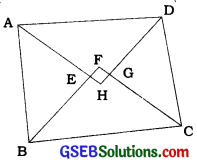In the given figure, ABCD is a quadrilateral in which the angle bisectors AH, BE CF and DH of internal angles A, B, C and D respectively form a quadrilateral EFGH.
Now, ∠FEH = ∠AEB
= 180° – ∠EAB – ∠EBA (Vertically opposite angles and angle sum property of triangle)
= 180°- j (∠A + ∠B)
and ∠FGH = ∠CGD
= 180° – ∠GCD – ∠GDC
(Vertically opposite angles and s angle sum property’of triangle)
= 180°- ^ (∠C + ∠D).

Then, ∠FEH + ∠FGH
= 180°- $$\frac{1}{2}$$(∠A + ∠B) + 180°- $$\frac{1}{2}$$(∠C + ∠ D) .
= 360° – $$\frac{1}{2}$$ (∠A + ∠B + ∠C + ∠D)
= 360°- $$\frac{1}{2}$$ × 360°
= 360°- 180° = 180° :
Hence, by theorem 10.12, quadrilateral EFGH is cyclic.